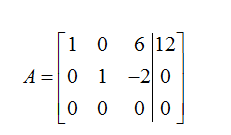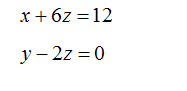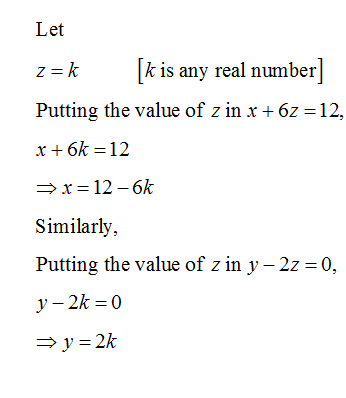# The augmented matrix below represents a system of linear equations associated with a real world prob-lem. The augmented matrix has already been completely row reduced.1   0    6  12 0   1  -2   00   0   0   0(a) Use the reduced matrix to write down the parametric solution for the system as a point (x, y, z).

Question
1 views

The augmented matrix below represents a system of linear equations associated with a real world prob-

lem. The augmented matrix has already been completely row reduced.

1   0    6  12

0   1  -2   0

0   0   0   0

(a) Use the reduced matrix to write down the parametric solution for the system as a point (x, y, z).

check_circle

Step 1

Let the given augmented matrix be:Step 2

Now,

The equation becomes,...

### Want to see the full answer?

See Solution

#### Want to see this answer and more?

Solutions are written by subject experts who are available 24/7. Questions are typically answered within 1 hour.*

See Solution
*Response times may vary by subject and question.
Tagged in

### Algebra